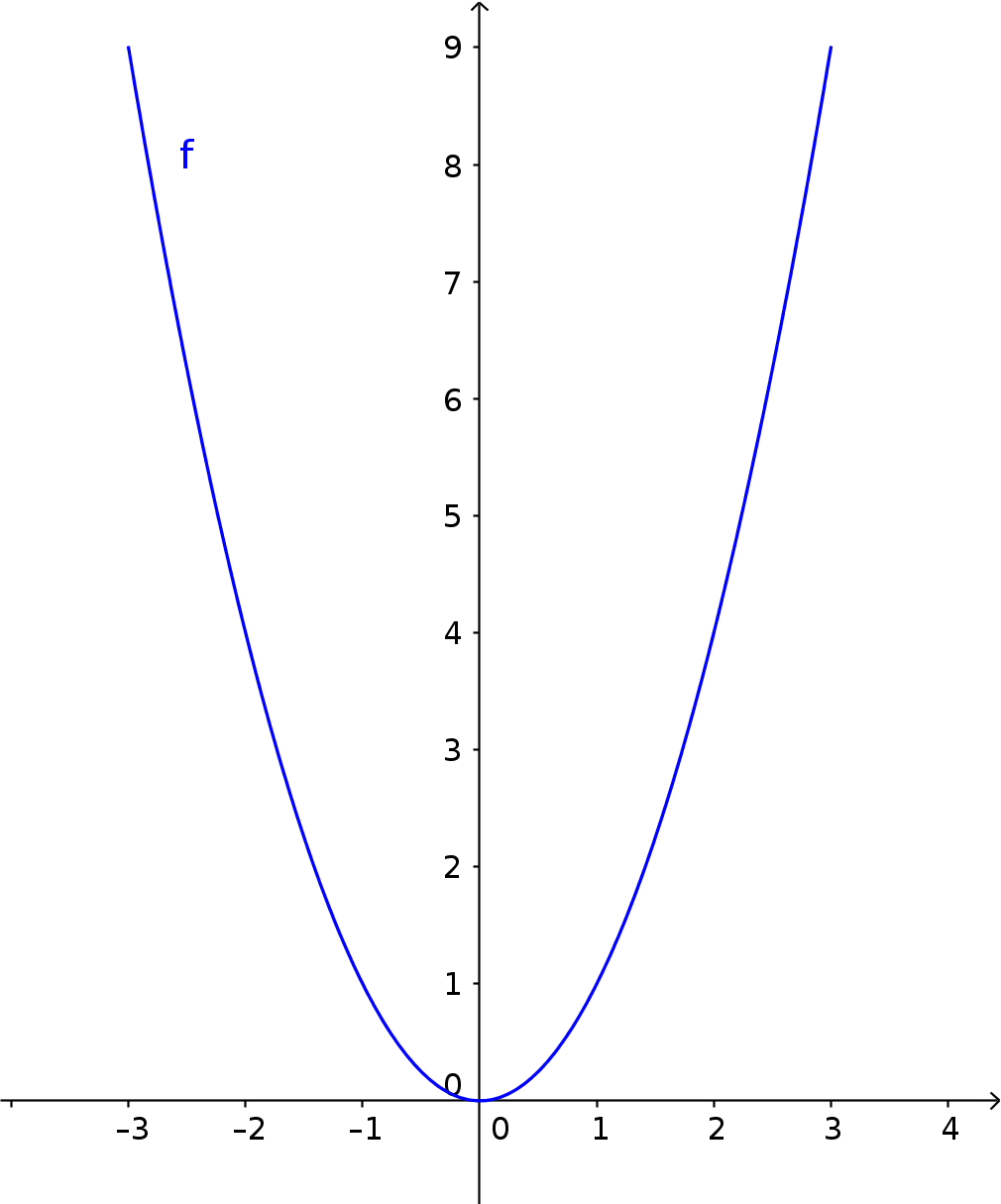Math Quadratic functions The standard parabola

# The standard parabola

The standard parabola is the simplest quadratic function. It has the function equation $y=x^2$

i

### Properties

• Graph symmetrical to the y-axis
• Coordinate origin ($0|0$) is the vertex and the lowest point of the graph
• Graph falls in the 2nd quadrant and rises in the 1st quadrant

### Example

Draw the standard parabola.

1. #### Create a value table

The standard parabola has the function equation $f(x)=x^2$. For $x$ we now use values from -3 to 3.

$f(-3)=(-3)^2=9$
$f(-2.5)=(-2.5)^2=6.25$
$f(-2)=(-2)^2=4$
...
$f(3)=3^2=9$
2. #### Enter values in a coordinate system